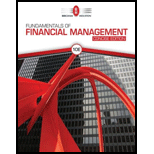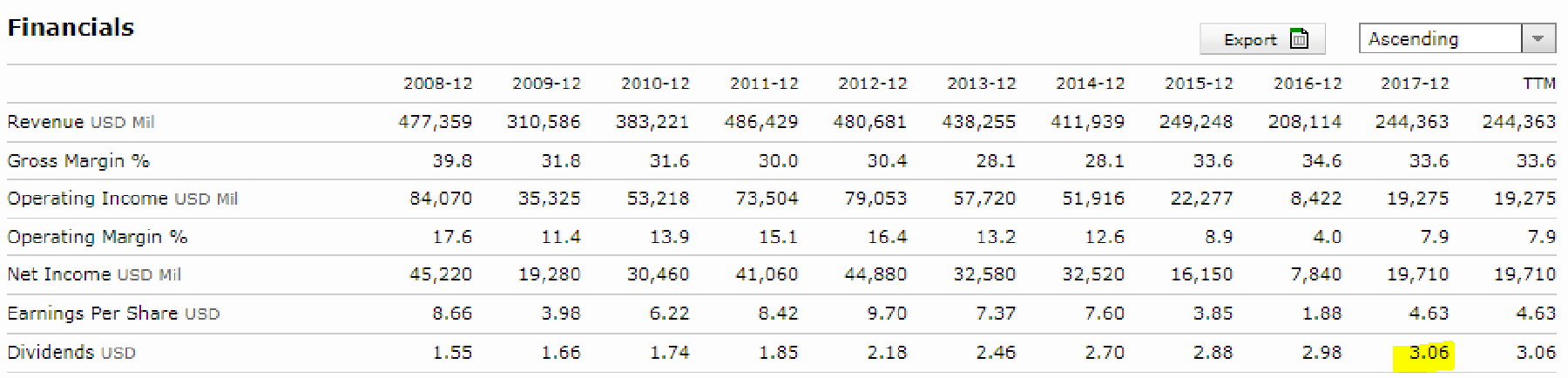Chapter 9, Problem 5TCL### Fundamentals Of Financial Manageme...

10th Edition
Eugene F. Brigham + 1 other
ISBN: 9781337902571

#### Solutions

Chapter
Section### Fundamentals Of Financial Manageme...

10th Edition
Eugene F. Brigham + 1 other
ISBN: 9781337902571
Textbook Problem

# In the text, we discussed using the discounted dividend model to estimate a stock’s intrinsic value. To keep things as simple as possible, let’s assume at first that XOM’s dividend is expected to grow at a constant rate of 5% annually over time. So, g = 5%. If so, the intrinsic value equals D1/(rs − g), where D1 is the expected annual dividend 1 year from now, rs is the stock’s required rate of return, and g is the dividend’s constant growth rate. Go back to the summary (overview) screen and find XOM’s current dividend. Multiply this dividend by 1 + g to arrive at an estimate of D1.Summary Introduction

To calculate: The amount of next year dividend per share.

Introduction:

Dividends:

It refers to the return over shares that is provided to the shareholders as a part of their earnings on the shares that they have invested in a company. It is that part of the total earnings that the company distributes among its shareholders.

Explanation

Given information:

Get As ASAP

#### Find more solutions based on key concepts